Search

Useful Lists

Partners

Online Manuals

Implement Bayesian inference using PHP, Part 1
By Paul Meagher - 2004-04-21 Page:  1 2 3 4 5 6 7 8 9 10 11

## Medical diagnosis wizard

To make it clear how Bayes theorem works, you will develop an online medical diagnosis wizard using PHP. This wizard could also have been called a calculator except that it takes four input steps to supply the prerequisite information then a step to review the result.

The wizard works by asking the user to supply the various pieces of information critical to computing the full posterior probability. The user can examine the posterior distribution to determine which which disease hypothesis enjoys the highest probability based on:

1. The diagnositic test information
2. The sample data used to estimate the prior and likelihood distributions

### Bayes Wizard: Step 1

Step 1 in using Bayes theorem to make a medical diagnosis involves specifying the number of disease alternatives that you will examine along with the number of symptoms or evidence keys. In the generic example you will look at, you will evaluate three disease alternatives based on evidence from two diagnostic tests. Each diagnostic test can only produce a positive or negative result. This means that the total number of symptom combinations, or evidence keys, you can observe is four (++, +-, -+, or --).

Figure 3. Form to enter disease hypotheses and symptom possibilities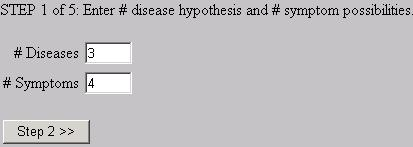### Bayes Wizard: Step 2

Step 2 involves entering the disease and symptom labels. In this case, you are just going to enter d1, d2, and d3 for the disease labels and ++, +-, -+ and -- for the symptom labels. The two symbols used for symptom labels signify whether the results of the two diagnostic tests came out positive or negative.

Figure 4. Form to enter disease and symptom labels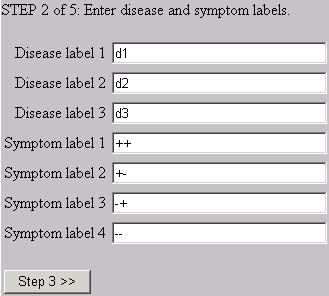### Bayes Wizard: Step 3

Step 3 involves entering the prior probabilities for each disease. You will use the data table below to determine the prior probabilities to enter for step three and the likelihood to enter for step four (this data table originally appeared in Introduction to Probability). Using this example allows you to confirm that the final result you obtain from the wizard agrees with the results you can find in this book.

Figure 5. Joint frequency of diseases and symptoms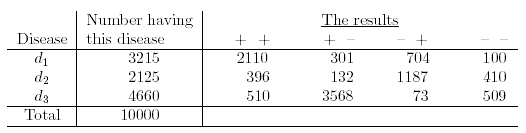The prior probability of each disease refers to the number of patients diagnosed with each disease divided by the total number of diagnosed cases in this sample. The relevant prior probabilities for each disease are entered in the following:

Figure 6. Form to enter disease priors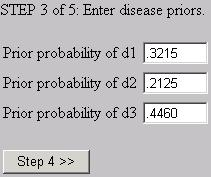You do not have to rely upon a data table such as the previous one to derive the prior probability estimates. In some cases, you can derive prior probabilities by using common-sense reasoning: The prior probability of a fair two-sided coin coming up heads is 0.5. The prior probability of selecting a queen of hearts from a randomized deck of cards is 1/52.

You also commonly run into situations where you intially have no good estimates of what the prior probability of each hypothesis might be. In such cases, it is common to posit noninformative priors. If you have four hypothesis alternatives, then the noninformative prior distribution would be 1/4 or 0.25 for each hypothesis. You might note here that Bayesians often criticize the use of a null hypothesis in significance testing because it amounts to assuming noninformative priors in cases where positing informative priors might be more theoretically or empirically justified.

A final way to derive estimates of the prior probability of each hypothesis P(Hi) is through a subjective estimate of what those probabilities might be given everything you have learned about the way the world works up to that point P( H=h | Everything you know). You will often find Bayesian inference sharing the same bed with a subjective view of probability in which the probability of a proposition is equated with one's subjective degree of belief in the proposition.

What it important in this discussion is that Bayesian inference is a flexible technique that allows you to estimate prior probabilities using objective methods, common-sense logical methods, and subjective methods. When using subjective methods, you must still be willing to defend your prior probability estimates. You may use objective data to help set and justify your subjective estimates which means that Bayesian inference is not necessarily in conflict with more objectively oriented approaches to statistical inference.

### Bayes Wizard: Step 4

The data table provides you with information you can use to compute the probability of the symptoms (like test results) given the disease, also known as the likelihood distribution P(E | H).

To see how the likelihood values entered below were computed, you can unpack P(E|H) using the frequency format for computing conditional probabilities:

P(E | H) = {E & H} / {H}

This tells us that you need to divide a joint frequency count {E & H} by a marginal frequency count {H} to obtain the likelihood value for each cell in your likelihood matrix. The top left cell of your likelihood matrix P(E='++' | H='d1) can be immediately computed from the joint and marginal frequency counts appearing in the data table:

P(E='++' | H='d1) = 2110 / 3125 = .6562

All the likelihood values entered in Step 4 were computed in this manner.

Figure 7. Form to enter likelihood of symptoms given the disease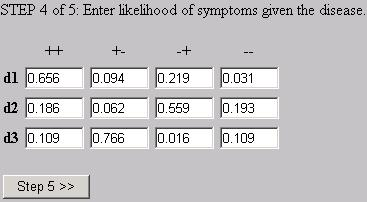It should be noted that many statisticians use likelihood as a system of inference instead of, or in addition to, Bayesian inference. This is because likelihoods also provide a metric one can use to evaluate the relative degree of support for several hypotheses given the data.

In the previous example, you can see that the probability of a particular evidence key varies for each hypothesis under consideration. The probability of the ++ evidence key is the greatest for the d1 hypothesis. You can assess which hypothesis is best supported by the data by:

1. Examining the likelihood of the evidence key given each hypothesis key
2. Selecting the hypothesis that maximizes the likelihood of the evidence key

Doing so would be an example of inference according to the principle of maximum likelihood.

Another interesting point to note is that all the values in the above likelihood distibution sum to a value greater than 1. What this means is that the likelihood distribution is not really a probability distribution because it lacks the defining property that the distribution of values sum to 1. This summation property is not essential for the purposes of evaluating the relative support for different hypotheses. What is important for this purpose is that the "likelihood supplies a natural order of preference among the possibilities under consideration" (from R.A. Fisher's Statistical Methods and Scientific Inference, p. 68).

You may not understand fully the concept of likelihood from this brief discussion, but I do hope that you appreciate its importance to the overall Bayes theorem calculation and its importance as the foundation for another system of inference. The likelihood system of inference is preferred by many statisticians because you don't have to resort to the dubious practice of trying to estimate the prior probability of each hypothesis.

Maximum likelihood estimators also have many desirable mathematical properties that make them nice to work with (the properties include transitivity, additivity, a lack of bias, and invariance under transformations, among others). For these reasons, it is often a good idea to closely examine your likelihood distribution in addition to your posterior distibution when making inferences from your data.

### Bayes Wizard: Step 5

The final step of the process involves displaying the posterior distribution of the diseases given the symptoms P(H | E):

Figure 8. Probability of each disease given symptoms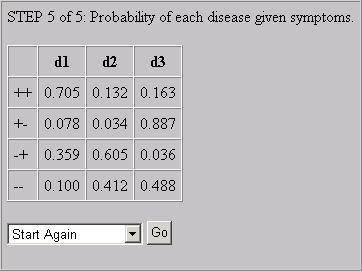The section of the script that was used to compute and display the posterior distribution looks like this:

Listing 4. Computing and displaying the posterior distribution
 ``````getPosterior(); \$bayes->setRowLabels(\$symptom_labels); // aka evidence labels \$bayes->setColumnLabels(\$disease_labels); // aka hypothesis labels \$bayes->toHTML(); ?>``````

You begin by loading the Bayes constructor with the priors and likelihoods obtained from previous wizard steps. Using this information, you compute the posterior using the `\$bayes->getPosterior()` method. To output the posterior distribution to the browser, you first set the row and column labels to display, then output the posterior distribution using the `\$bayes->toHTML()` method.

View Implement Bayesian inference using PHP, Part 1 Discussion

Page:  1 2 3 4 5 6 7 8 9 10 11